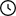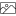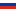• RU
•Waiting For Moderation: 29

# Design of electric locomotive depot foundations - course

• Size: 1 MB

## Description

Two options are considered - shallow foundations and piles, on the basis of calculations a choice was made about the best option calculated in the course design

## Project's Contentmgiof.rar [ 1 MB ]Лист.dwg [ 512 KB]Титульник.docx [ 14 KB]записка .docx [ 1 MB]

### Contents

Contents

Introduction

Source data. Assessment of geotechnical conditions

platforms

Purpose and structural features of underground part

buildings

Site characteristics, geotechnical and

hydrogeological conditions

Site Soil Construction Classification

Assessment of construction properties of site soils and possible

building foundation options

Foundations of shallow laying

Select the type and construction of foundations. Assigning Depth

laying of foundations

Loads taken into account in foundation base calculations

Sizing the Foundation Floor

Check base stresses and refine sole dimensions

base

Foundation settlement calculation

Calculation of foundation settlement in time

Piling foundation variant

Selection of type and structure of piles and pile foundation

Assignment of pile pile depth

Determination of pile bearing capacity and design load,

basis allowed for pile and material strength

piles

Determination of the number of piles in the foundation. Checking actual

Settlement calculation of pile foundation

Compare Foundation Options and Select Main

Calculation of quantities of work and calculation of cost of one device

base

Conclusion

Literature

### Design of electric locomotive depot foundations

Introduction

In this course design, in the same soil conditions and for the same structure, different types of foundations will be proposed, from which you need to choose the most rational, guaranteeing the strength and stability of the structures. foundation calculation will be carried out for the electric locomotive depot.

### Initial data and assessment of engineering and geological conditions of the construction site

1. Purpose and structural features of the underground part of the building

elevation of natural relief surface NL = 130.0 m;

standard ground freezing depth dfn = 1.4 m.

The assessment of the engineering and geologic conditions of the site begins with the study of soil stratum. To do this, according to the initial data (Table 1), we construct a geological section (Figure 2).

1.2. Site characteristics, geotechnical and hydrogeological conditions

To substantiate the design solutions for the foundation arrangement, it is necessary, first of all, to study the engineering and geologic conditions of the site.

Assessment of the engineering and geological conditions of the site begins with the study of soil stratum, for this a geological section is built.

To quantify the strength and deformation properties of the soil of the site, we calculate derivative characteristics of the physical properties of the soil, which include:

a) for sandy soils - porosity coefficient and degree of importance;

b) for dusty-clay soils - ductility number, yield index, porosity coefficient and humidity degree.

It is necessary to find out whether dusty-clay soils belong to subsidence or swelling. For this, you can limit yourself to an approximate estimate of the leakage and swelling of soils. Subsidence soils include clay soils with a degree of humidity Sr≤0,8, for which the value of the Iss index, determined by the formula:

less than: 0.1 at; 0.17 at and 0.24 at. Water swellable from soaking includes clay soils, for which the value of the Iss≥0,3 indicator is.

In the formula eL - the porosity coefficient corresponding to humidity at the flow boundary wL, determined by the formula:

We determine the degree of moisture of loam:

Since Sr > 0.8, therefore, further verification is not carried out - loam is not leaking or swelling.

Plasticity Number:

By the number of plasticity - clay;

Yield Index:

According to the yield index, clay is refractory.

We check whether clay is related to subsidence or swelling soils.

We determine the degree of moisture of the clay:

Since Sr > 0.8 is therefore not further checked - clay is not subsident or swellable.

Plasticity Number:

By the number of plasticity - soup;

Yield Index:

In terms of fluidity, the soup is plastic.

We check whether the soup belongs to subsidence or swelling soils.

We determine the degree of moisture of the clay:

Since Sr > 0.8, therefore, we do not carry out further verification - the slurry is not sedimentary or swelling.

Porosity coefficient (ratio of pore volume to soil particle volume):

By porosity coefficient - medium-density sand;

Degree of soil humidity:

By degree of humidity (degree of filling of pores with water) - sand saturated with water;

According to the values ​ ​ of the characteristics of the physical properties of soils, which determine their type and variety, the value of the internal friction angle sound, specific adhesion with, deformation modulus E and design resistance R0 are written out from the corresponding tables.

Assessment of the engineering and geological conditions of the site begins with the construction of a geological section, on which, in addition to soil layers, the level of underground water is applied, and its elevation is fixed (Figure 2).

1.3. Site Soil Construction Classification

The basis for the construction of the building is sandy and clay soils. Sandy soils according to the granulometric composition are divided into the following types: gravelly sand, coarse sand, medium-sized sand, fine sand, dusty sand.

By the degree of humidity, sandy soils are divided into varieties: low-moisture, wet, saturated with water.

On plasticity number soil is subdivided on: sandy loam, loam, clay.

According to the yield indicator, dusty-clay soils are divided: soup (hard, plastic, fluid), loams and clays (hard, semi-solid, refractory, soft-plastic, fluid-plastic, fluid-flexible).

1.4. Assessment of construction properties of site soils and possible variants of building foundations

The characteristics of the mechanical properties of soils (¼, c, E) and the value of the design resistance R0 can be judged by the bearing capacity, deformability of the soil and the possibility of using it as a base.

In the design, all soils belong to low-pressure (E 20MPa) and medium-pressure (20 > E 5MPa), so all of them can be used as bases for capital buildings.

If R0 < 100kPa, then the issue of using such soil as a base can be resolved only on the basis of research.

In the design, all soils have design resistance R0 > 100kPa, thus they can be used as foundations.

Since the base soils have sufficient bearing capacity, shallow foundations and pile foundations can be used.

### Foundations of shallow laying

2.1. Select the type and construction of foundations. Purpose of foundation depth

The type of foundation is chosen depending on the nature of the transfer of load to the foundation: tape foundations of prefabricated elements are usually arranged for the walls of the building, and individual glass-type foundations for prefabricated reinforced concrete columns.

The depth of the foundation depends on many factors. The determining ones are:

- geotechnical and hydrogeological conditions of the site are the position of the bearing soil layer;

- depth of ground freezing, if there are heavy soils in the base;

- structural features of the underground part of the building (presence of a basement, communication, adjoining the neighboring building, etc.).

Depth of laying of foundations of external walls and columns taking into account freezing depth is assigned in accordance with the instructions given in Table 2 of SNiP. The calculated depth of seasonal freezing of soil is determined by the formula:

We accept: for a production building (according to the task - scheme No. 7 electric locomotive depot) without a basement with floors arranged on the ground, coefficient kh = 0.6

From where the estimated depth of seasonal freezing of the soil df near the foundation is determined:

Accept depth of laying df = 1.5 (standard freezing depth is taken according to dfn = 1,4 m):

d > df

2.2 Loads taken into account in foundation base calculations

In accordance with SNiP , the bases of foundations folded with non-rock soils are calculated according to the limit state of the second group, i.e., by deformations.

In the course design, the normative values ​ ​ of loads and impacts in the plane of cutting the foundation of the building are given in the initial data. Values of design loads and impacts for calculation by deformations are taken equal to the normative one (αf = 1.0), for calculation by bearing capacity - by multiplying the standard loads by the averaged reliability factor for loads αf = 1.2.

2.3. Sizing the Foundation Floor

The dimensions of the foundation foot depend on a number of related parameters and are established by sequential approximation. In the first approximation, the sole area:

2.4 Check of stress in the base and clarification of dimensions of the foundation foot

Dimensions of foundation sole adopted in the first approach as per

We determine the average pressure under the foundation base and the minimum value of the edge pressure:

The difference between p and R is 19.2%. The size of the foundation foot for the external wall of the building is correct.

We finally accept the foundation 3 with dimensions of 2.4 x 1.5 m.

2.5 Calculation of foundation settlement

Value of final settlement is determined by method of layer-by-layer summation of op formula

The obtained settlement value is compared with the maximum permissible value established by the design standards.

Settlement calculation is performed in the following sequence:

1) the base thickness is divided into layers within a certain limited depth (4 times the width of the foundation base). Thickness of layer is taken as 0.4 of foundation width ().

2) calculate the vertical stress values ​ ​ of the own weight of the soil at the boundaries of the selected layers along the Z axis passing through the center of the foundation sole by the formula:

The specific gravity of the soil lying below the level of groundwater, but above the water stop, is taken taking into account the weighing effect of the water. The pressure of the water column should be taken into account when determining αzg in the water-resistant layer;

3) determine additional vertical stresses at the boundaries of the selected layers along the Z axis passing through the center of the foundation base by the formula:

5) the lower boundary of the compressible thickness of the base, set at the depth where the condition is met

6) calculate the deformation values ​ ​ of each layer of the compressible thickness, and then determine the foundation settlement by summing up the deformations of individual layers.

Sole width b = 2.4 m, average pressure under foundation base:

Stresses due to soil dead weight at the foundation bottom level:

The results of the sediment calculation are summarized in Table 3

The obtained settlement value s = 5.68cm is less than the maximum permissible settlement.

Calculation of foundation settlement 3:

Sole width b = 1.5 m, average pressure under foundation base:

stresses due to soil dead weight at the level of foundation base:

The results of the sediment calculation are summarized in Table 4

2.6 Calculation of foundation settlement over time

In this course project, the foundation settlement in time is calculated for only one foundation 1 according to the methodology based on the solution of the one-dimensional problem of linear filtration consolidation.

We calculate the consolidation of the base of the tape foundation with a base width of b = 2.4 m, a laying depth of d = 2.0 m. Under the base of the foundation lies a clay bed with a thickness of h = 4.0 m. The final foundation sediment due to the compaction of clays s = 5.68 cm. Filtration coefficient kf = 5⋅108 cm/s = 1.5 cm/year = 0.015 m/year.

Relative compressibility factor:

### Piling foundation variant

3.1 Selection of type and structure of piles and pile foundation.

Assignment of pile pile depth

We accept typical prismatic reinforced concrete piles. The type of foundation is pile bushes for the columns of the frame building, consisting of a group of piles and a low pile pile, in combination with foundation beams.

The pedestals of the baseless building can be laid at the ground surface with a depth of 0.1-0.2 m below the layout surface, but a layer of slag with a thickness of at least 0.3 m should be laid under the pedestals.

For foundation 1:

Since the dimensions of the sub-column for the column are 1.2x1.2x2.1, and the sub-column is buried in the ground up to 0.15 m from the ground surface and taking the height of the pedestal equal to 0.4 m then, we accept the depth of the base of the pedestal:

We accept pile C730 with the following characteristics:

• concrete M 200;

• pile weight 1.6 t;

• longitudinal reinforcement 4 ∅ 14S240;

pile section 300300mm.

We accept pile C730 with the following characteristics:

• concrete M 200;

• pile weight 1.6 t;

• longitudinal reinforcement 4 ∅ 14S240;

pile section 300300mm.

3.2 Determination of pile bearing capacity and design load allowed on pile by base soil and strength of pile material

The bearing capacity of the pile is determined by the formula:

3.3 Determination of the number of piles in the foundation. Verification of actual load transferred to pile

The number of piles in the pile foundation is calculated according to the limit state of the first group. For this purpose design loads and impacts are determined by multiplying standard forces by averaged reliability factor taken equal to 1.2.

The number of piles in the pile foundation for the column is determined as follows:

and a) calculating the mean pressure under the base of the pile by taking the distance between the axes of the piles 3d from the expression:

At that, weight of pile pile with soil on ledges is determined by:

3.4 Calculation of pile foundation settlement

The expected settlement of the pile foundation from hanging piles is determined by calculating the limit states of the second group. We will calculate the settlement of the foundation, considering the pile foundation as a conditional massif.

Side faces of the conditional pattern are spaced from the faces of the pile at a distance of ^

l = htg(ϕII,mt/4);

We calculate the design failure of the pile.

Pile weight with cap Gc = 20 + 1 = 21 kN. We choose the type of hammer based on the condition of the ratio of the weight of the impact part and the weight of the pile with the head. When the pile is immersed in the ground, the weight of the hammer impact part must be at least G=1,25⋅Gc=1,25⋅21=26,25kN. When submerged with a tubular diesel hammer G=0,7Gc=0,7⋅21=14,7kN. We preselect a tubular diesel hammer S-996and with a 25 kN impact weight, impact energy at a height of 2.5 m 27 kJ, a hammer weight with a cat of 3.65 tons.

Design value of impact energy for tubular diesel hammers:

We calculate the design failure of the pile by the formula:

### Compare Foundation Options and Select Main

4.1 Calculation of the scope of work and calculation of the cost of one foundation

Foundation 1

The main option is selected by comparing the cost of the foundation structure on a natural base with the cost of the pile foundation structure. To simplify the calculation of the scope of work, Figures 11 and 12 show sketches of a separate glass-type foundation and a pile foundation, respectively

Based on the calculated cost of the designed foundations, we accept the pile foundation as the main option.

Foundation 3

The main option is selected by comparing the cost of the foundation structure on a natural base with the cost of the pile foundation structure. To simplify the calculation of the scope of work, Figures 13 and 14 show sketches of a separate glass-type foundation and a pile foundation, respectively

Based on the calculated cost of the designed foundations, we accept the pile foundation as the main option.

Conclusion

In this course project, separate foundations for the electric locomotive depot building are considered. Based on the calculations and comparison of the costs of the foundation device on a natural base and the costs of the pile foundation device, it was determined that the most expedient use of pile separate foundations for the column.

### Literature

1. Foundations and foundations of buildings and structures. SNB 5.01.0199.

07.01.1999.-M: Ministry of Construction and Architecture of the Republic of Belarus, 1999.

2. Pile foundations. SNiP 2.02.0385; 01.01.1985.-M: NIIOSP named after Gersevanov Gosstroy of the USSR, 1995

3. Votyakov, I.F. Soil mechanics, foundations and foundations: Methodological instructions. - Gomel, 1996. – 60s

4. Kudryavtsev, I.A. Foundations and Foundations: Manual/I.A. Kudryavtsev, K.N. Pirogovsky. Gomel: BelGUT, 2003.306 p.

5. Reinforced Concrete Foundation Slabs: Specification.

GOST 1358085; 01.01.1987.-M: State Committee for Civil Engineering and Architecture at the USSR Gosstroy, 1985.

6. Concrete blocks for basement walls: Specification. GOST 1357978;

01.01.1978.-M: State Committee for Civil Engineering and Architecture at the USSR Gosstroy, 1978

Drawings contentЛист.dwg